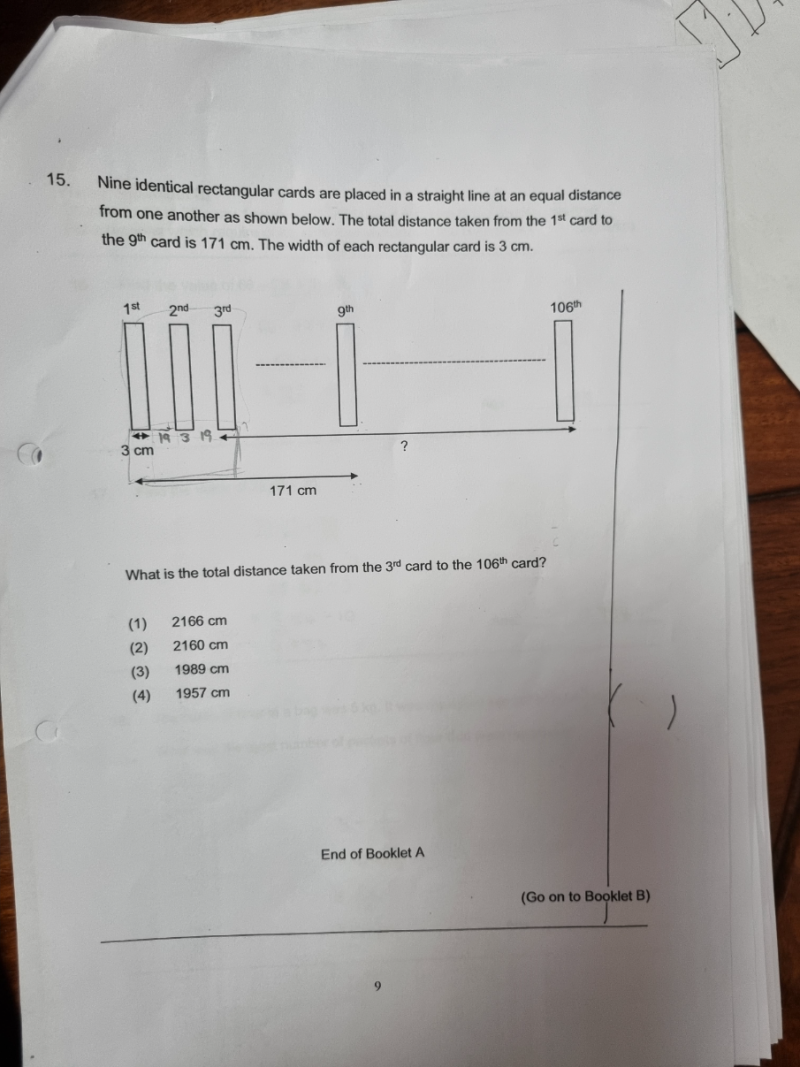# QuestionPls help thanks

9 x 3 = 27

171 – 27 = 144

9 cards have 8 spaces in between the cards, so

Each space = 144/8 = 18

Solution 1 (Calculate the distance from 3rd to 106th card) directly):

Number of cards from 3rd to 106th = 106 – 3 + 1 = 104

104 x 3 = 312

104 cards have 103 spaces between the cards. so

18 x 103 =  1854

Therefore, total distance from 3rd card to 106th card = 312 + 1854 = 2166 cm

Solution 2 (Calculate the distance from 1st to 106th and minus the 1st 2 cards’ distance b4 3rd card):

106 x 3 = 318

Distance from 1st to 106th card = 318 + (18 x 105) =  2208

Distance from 1st to just before 3rd card = 3 + 18 + 3 + 18 = 42

Therefore, distance from 3rd card to 106th card = 2208 – 42 = 2166 cm

PS: Whether Solution 1 or Solution 2, the pink part of the solution is the same for both solutions.

0 Replies 2 Likes ✔Accepted Answer